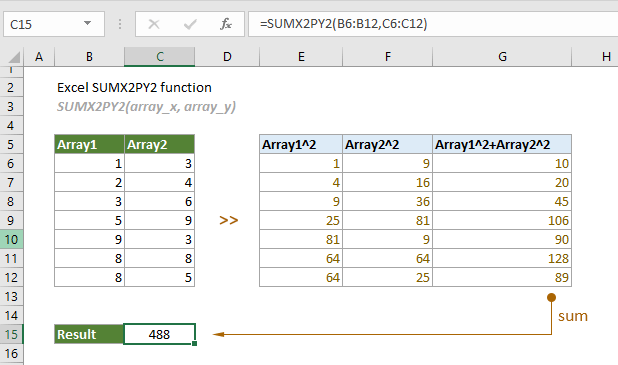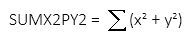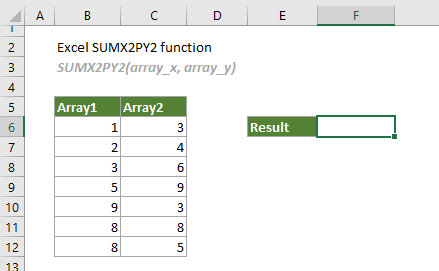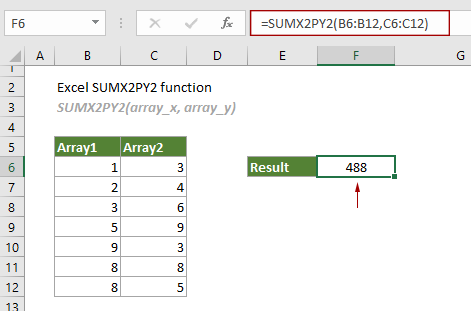## Excel SUMX2PY2 function

The SUMX2PY2 function returns the sum of squares of corresponding values in two given arrays.#### Syntax

SUMX2PY2(array_x, array_y)

#### Arguments

• Array_x (required): The first array or range of values used in the calculation;
• Array_y (required): The second array or range of values used in the calculation.

#### Remarks

1.Either of the following elements can be used in the supplied arguments:
-- Numbers;
-- Names, arrays or references that contain numbers.
2. If there are text, logical values or empty cells in the supplied array, they will be ignored;
3. Zero values will be included in the calculation；
4. The array_x and array_y must contain exactly the same number of values, otherwise, the #N/A! error value will be returned;
5. The equation of the SUMX2PY2 function is as follows:#### Return value

It returns a numeric value.

#### Example

As shown in the screenshot below, to get the sum of squares of array1 and array2, you can easily apply the SUMX2PY2 function to handle it.1. Select a blank cell, copy or enter the formula below and press the Enter key to get the result.

=SUMX2PY2(B6:B12,C6:C12)Notes:

1. The above result can be broken down as:

=(1^2+3^2)+(2^2+4^2)+(3^2+6^2)+(5^2+9^2)+(9^2+3^2)+(8^2+8^2)+(8^2+5^2)

2. You can directly use the cell values in the formula as follows.

=SUMX2PY2({1,2,3,5,9,8,8},{3,4,6,9,3,8,5})

#### Related Functions

Excel SUMX2MY2 function
The SUMX2MY2 function returns the sum of the difference of squares of corresponding values in two given arrays.

Excel SUMXMY2 function
The SUMXMY2 function returns the sum of squares of the differences of corresponding values in two given arrays.

### The Best Office Productivity Tools

#### Kutools for Excel - Helps You To Stand Out From Crowd

 Popular Features: Find, Highlight or Identify Duplicates  |  Delete Blank Rows  |  Combine Columns or Cells without Losing Data  |  Round without Formula ... Super VLookup: Multiple Criteria  |  Multiple Value  |  Across Multi-Sheets  |  Fuzzy Lookup... Adv. Drop-down List: Easy Drop Down List  |  Dependent Drop Down List  |  Multi-select Drop Down List... Column Manager: Add a Specific Number of Columns  |  Move Columns  |  Toggle Visibility Status of Hidden Columns  |  Compare Columns to Select Same & Different Cells ... Featured Features: Grid Focus  |  Design View  |  Big Formula Bar  |  Workbook & Sheet Manager | Resource Library (Auto Text)  |  Date Picker  |  Combine Worksheets  |  Encrypt/Decrypt Cells  |  Send Emails by List  |  Super Filter  |  Special Filter (filter bold/italic/strikethrough...) ... Top 15 Toolsets:  12 Text Tools (Add Text, Remove Characters ...)  |  50+ Chart Types (Gantt Chart ...)  |  40+ Practical Formulas (Calculate age based on birthday ...)  |  19 Insertion Tools (Insert QR Code, Insert Picture from Path ...)  |  12 Conversion Tools (Numbers to Words, Currency Conversion ...)  |  7 Merge & Split Tools (Advanced Combine Rows, Split Excel Cells ...)  |  ... and more

Kutools for Excel Boasts Over 300 Features, Ensuring That What You Need is Just A Click Away...#### Office Tab - Enable Tabbed Reading and Editing in Microsoft Office (include Excel)

• One second to switch between dozens of open documents!
• Reduce hundreds of mouse clicks for you every day, say goodbye to mouse hand.
• Increases your productivity by 50% when viewing and editing multiple documents.
• Brings Efficient Tabs to Office (include Excel), Just Like Chrome, Edge and Firefox.